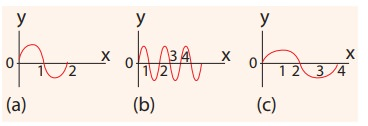Home | | Physics 11th std | Solved Example Problems for Wave Motion

# Solved Example Problems for Wave Motion

Physics : Waves - Solved Example Problems for Wave Motion

EXAMPLE 11.1

Which of the following has longer wavelength?### EXAMPLE 11.2

Three waves are shown in the figure belowWrite down

(a) the frequency in ascending order

(b) the wavelength in ascending order

### Solution

(a) fc < fa < fb

(b) λb < λa < λc

From the example 11.2, we observe that the frequency is inversely related to the wavelength, f ∝ 1/λ

Then, fλ is equal to what?

[(i.e) fλ=?]

A simple dimensional argument will help us to determine this unknown physical quantity.

Dimension of wavelength is, [λ] = L

Frequency f = 1/ Time period , which implies that the dimension of frequency is,where v is known as the wave velocity or phase velocity. This is the velocity with which the wave propagates. Wave velocity is the distance travelled by a wave in one second.

Note:

1. The number of cycles (or revolutions) per unit time is called angular frequencyAngular frequency, ω = 2π/T = 2πf (unit is radians/second)

2. The number of cycles per unit distance or number of waves per unit distance is called wave number.

wave number, k = 2π/λ (unit is radians/ meter)

In two, three or higher dimensional case, the wave number is the magnitude of a vector called wave vector. The points in space of wave vectors are called reciprocal vectors,.

Dimensions ofis L-1.

The velocity v, angular frequency ω and wave number λ are related as:Wave numbers and wave vectors play an essential role in optics and scattering theory.

### EXAMPLE 11.3

The average range of frequencies at which human beings can hear sound waves varies from 20 Hz to 20 kHz. Calculate the wavelength of the sound wave in these limits. (Assume the speed of sound to be 340 m s-1.

### SolutionTherefore, the audible wavelength region is from 0.017 m to 17 m when the velocity of sound in that region is 340 m s-1.

### EXAMPLE 11.4

A man saw a toy duck on a wave in an ocean. He noticed that the duck moved up and down 15 times per minute. He roughly measured the wavelength of the ocean wave as 1.2 m. Calculate the time taken by the toy duck for going one time up and down and also the velocity of the ocean wave.### Solution

Given that the number of times the toy duck moves up and down is 15 times per minute. This information gives us frequency (the number of times the toy duck moves up and down)But one minute is 60 second, therefore, expressing time in terms of secondThe time taken by the toy duck for going one time up and down is time period which is inverse of frequencyThe velocity of ocean wave is v

v = λf = 1.2 ×0.25 = 0.3 m s-1.

### EXAMPLE 11.5

Consider a string whose one end is attached to a wall. Then compute the following in both situations given in figure (assume waves crosses the distance in one second)(a)  Wavelength,

(b)  Frequency and

(c)  Velocity

### SolutionThis means that the speed of the wave along a string is a constant. Higher the frequency, shorter the wavelength and vice versa, and their product is velocity which remains the same.

Tags : Physics , 11th Physics : UNIT 11 : Waves
Study Material, Lecturing Notes, Assignment, Reference, Wiki description explanation, brief detail
11th Physics : UNIT 11 : Waves : Solved Example Problems for Wave Motion | Physics